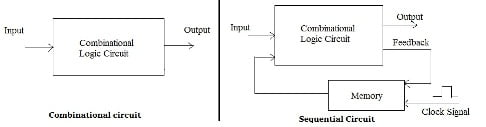# Difference between combinational and sequential circuitsDigital logic circuits can be classified into “combinational” and “sequential.” A combinational logic circuit is one whose output solely depends on its current inputs. Sequential circuits, on the other hand, are built using combinational circuit and memory elements called “flip-flops”. These circuits generate output which depends on the current and previous states.

## Combinational Circuits

A combinational circuit is one where the output at any time depends only on the present combination of inputs at that point of time with total disregard to the past state of the inputs. The logic gate is the most basic building block of combinational logic. The logical function performed by a combinational circuit is fully defined by a set of Boolean expressions.

A combinational circuit consists of n input variables and m output variables or simply outputs. Since the number of input variables is n, there are 2n possible combinations of bits at the input. If m is the number of output variable then there are m boolean functions corresponding to each output.

A block diagram of combinational circuit is shown in figure below.

## Sequential Circuits

Sequential circuit is a combination of a combinational circuit and a memory elements connected in feedback path. The memory elements are devices capable of storing binary information within them.The binary information stored in the memory elements at any given time defines the state of the sequential circuit. The sequential circuit receives binary information from external inputs. Thus, a sequential circuit is specified by a time sequence by a time sequence of inputs, outputs and internal states.

A block diagram of sequential circuit is shown in figure below.